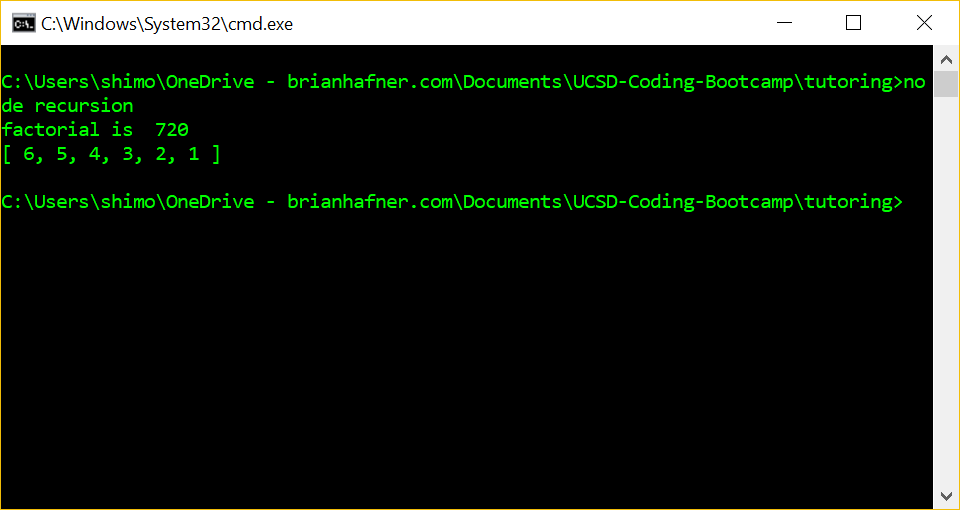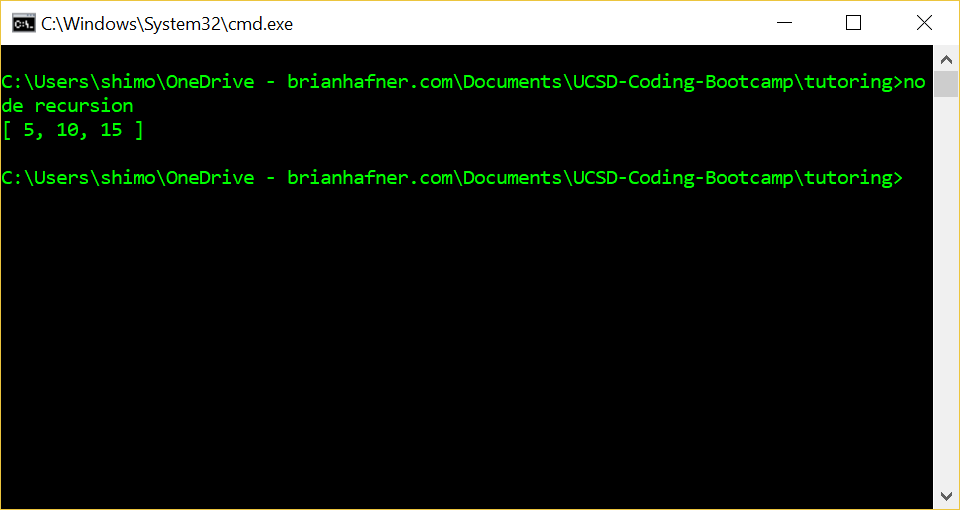## Javascript Recursion

I’m working on some problems to help me better understand recursion.  First, I will do a function to calculate n factorial (n!). This is how Wikipedia explains factorial:

In mathematics, the factorial of a non-negative integer n, denoted by n!, is the product of all positive integers less than or equal to n. For example,https://en.wikipedia.org/wiki/Factorial

The example below is a good case for using a recursive solution:

The trouble I had with recursion was in understanding where it is stored the product of the multiplication on line 5.  It turned out that it was being stored in a stack.  As I now understand it, I can think of the stack as an array.  Below I’ve added a stack array to illustrate this.Next I did a recursive function to calculate multiples of a number.  The function takes in base and i (the number of multiples to calculate).  The results are stored in the multiples array.